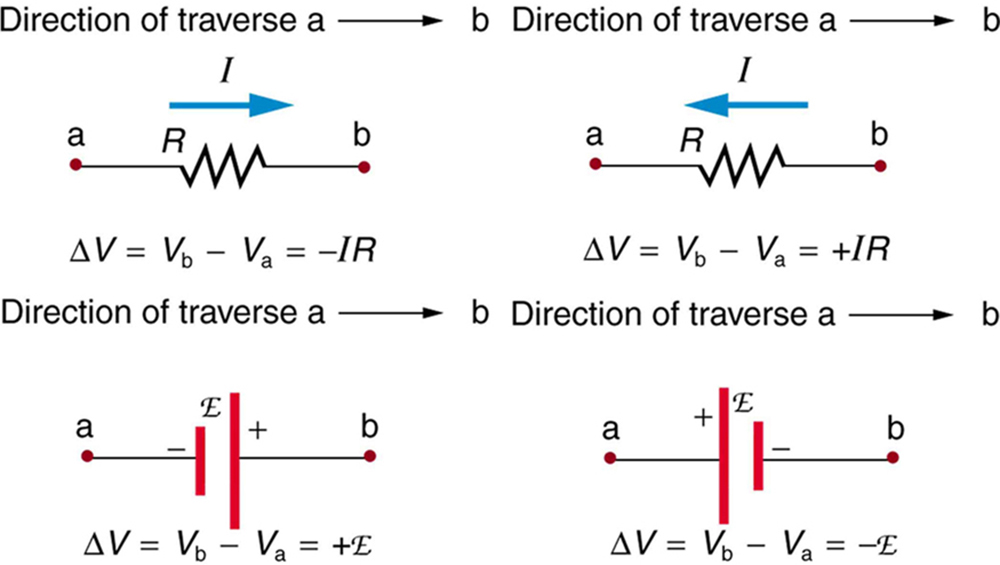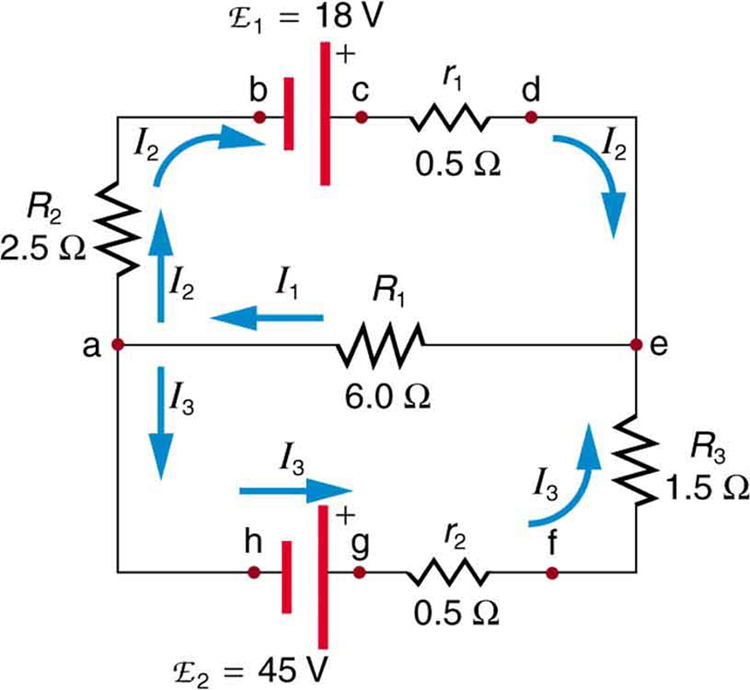# 21.3 Kirchhoff’s rules  (Page 3/10)

 Page 3 / 10

## Applying kirchhoff’s rules

By applying Kirchhoff’s rules, we generate equations that allow us to find the unknowns in circuits. The unknowns may be currents, emfs, or resistances. Each time a rule is applied, an equation is produced. If there are as many independent equations as unknowns, then the problem can be solved. There are two decisions you must make when applying Kirchhoff’s rules. These decisions determine the signs of various quantities in the equations you obtain from applying the rules.

1. When applying Kirchhoff’s first rule, the junction rule, you must label the current in each branch and decide in what direction it is going. For example, in [link] , [link] , and [link] , currents are labeled ${I}_{1}$ , ${I}_{2}$ , ${I}_{3}$ , and $I$ , and arrows indicate their directions. There is no risk here, for if you choose the wrong direction, the current will be of the correct magnitude but negative.
2. When applying Kirchhoff’s second rule, the loop rule, you must identify a closed loop and decide in which direction to go around it, clockwise or counterclockwise. For example, in [link] the loop was traversed in the same direction as the current (clockwise). Again, there is no risk; going around the circuit in the opposite direction reverses the sign of every term in the equation, which is like multiplying both sides of the equation by $–1.$

[link] and the following points will help you get the plus or minus signs right when applying the loop rule. Note that the resistors and emfs are traversed by going from a to b. In many circuits, it will be necessary to construct more than one loop. In traversing each loop, one needs to be consistent for the sign of the change in potential. (See [link] .)Each of these resistors and voltage sources is traversed from a to b. The potential changes are shown beneath each element and are explained in the text. (Note that the script E stands for emf.)
• When a resistor is traversed in the same direction as the current, the change in potential is $-\text{IR}$ . (See [link] .)
• When a resistor is traversed in the direction opposite to the current, the change in potential is $+\text{IR}$ . (See [link] .)
• When an emf is traversed from $–$ to + (the same direction it moves positive charge), the change in potential is +emf. (See [link] .)
• When an emf is traversed from + to $–$ (opposite to the direction it moves positive charge), the change in potential is $-$ emf. (See [link] .)

## Calculating current: using kirchhoff’s rules

Find the currents flowing in the circuit in [link] .This circuit is similar to that in [link] , but the resistances and emfs are specified. (Each emf is denoted by script E.) The currents in each branch are labeled and assumed to move in the directions shown. This example uses Kirchhoff’s rules to find the currents.

Strategy

This circuit is sufficiently complex that the currents cannot be found using Ohm’s law and the series-parallel techniques—it is necessary to use Kirchhoff’s rules. Currents have been labeled ${I}_{1}$ , ${I}_{2}$ , and ${I}_{3}$ in the figure and assumptions have been made about their directions. Locations on the diagram have been labeled with letters a through h. In the solution we will apply the junction and loop rules, seeking three independent equations to allow us to solve for the three unknown currents.

Determine the total force and the absolute pressure on the bottom of a swimming pool 28.0m by 8.5m whose uniform depth is 1 .8m.
for the answer to complete, the units need specified why
That's just how the AP grades. Otherwise, you could be talking about m/s when the answer requires m/s^2. They need to know what you are referring to.
Kyle
Suppose a speck of dust in an electrostatic precipitator has 1.0000×1012 protons in it and has a net charge of –5.00 nC (a very large charge for a small speck). How many electrons does it have?
how would I work this problem
Alexia
how can you have not an integer number of protons? If, on the other hand it supposed to be 1e12, then 1.6e-19C/proton • 1e12 protons=1.6e-7 C is the charge of the protons in the speck, so the difference between this and 5e-9C is made up by electrons
Igor
what is angular velocity
angular velocity can be defined as the rate of change in radian over seconds.
Fidelis
Why does earth exert only a tiny downward pull?
hello
Islam
Why is light bright?
an 8.0 capacitor is connected by to the terminals of 60Hz whoes rms voltage is 150v. a.find the capacity reactance and rms to the circuit
thanks so much. i undersooth well
what is physics
is the study of matter in relation to energy
Kintu
physics can be defined as the natural science that deals with the study of motion through space,time along with its related concepts which are energy and force
Fidelis
a submersible pump is dropped a borehole and hits the level of water at the bottom of the borehole 5 seconds later.determine the level of water in the borehole
what is power?
power P = Work done per second W/ t. It means the more power, the stronger machine
Sphere
e.g. heart Uses 2 W per beat.
Rohit
A spherica, concave shaving mirror has a radius of curvature of 32 cm .what is the magnification of a persons face. when it is 12cm to the left of the vertex of the mirror
did you solve?
Shii
1.75cm
Ridwan
my name is Abu m.konnek I am a student of a electrical engineer and I want you to help me
Abu
the magnification k = f/(f-d) with focus f = R/2 =16 cm; d =12 cm k = 16/4 =4
Sphere
what do we call velocity
Kings
A weather vane is some sort of directional arrow parallel to the ground that may rotate freely in a horizontal plane. A typical weather vane has a large cross-sectional area perpendicular to the direction the arrow is pointing, like a “One Way” street sign. The purpose of the weather vane is to indicate the direction of the wind. As wind blows pa
hi
Godfred
Godfred
If a prism is fully imersed in water then the ray of light will normally dispersed or their is any difference?
the same behavior thru the prism out or in water bud abbot
Ju
If this will experimented with a hollow(vaccum) prism in water then what will be result ?
AnuragByByByBy Brooke DelaneyBy Donyea SweetsBy Anonymous UserBy Richley CrapoBy LaToya TrowersBy Danielle StephensBy OpenStaxBy Inderjeet BrarBy Brooke Delaney